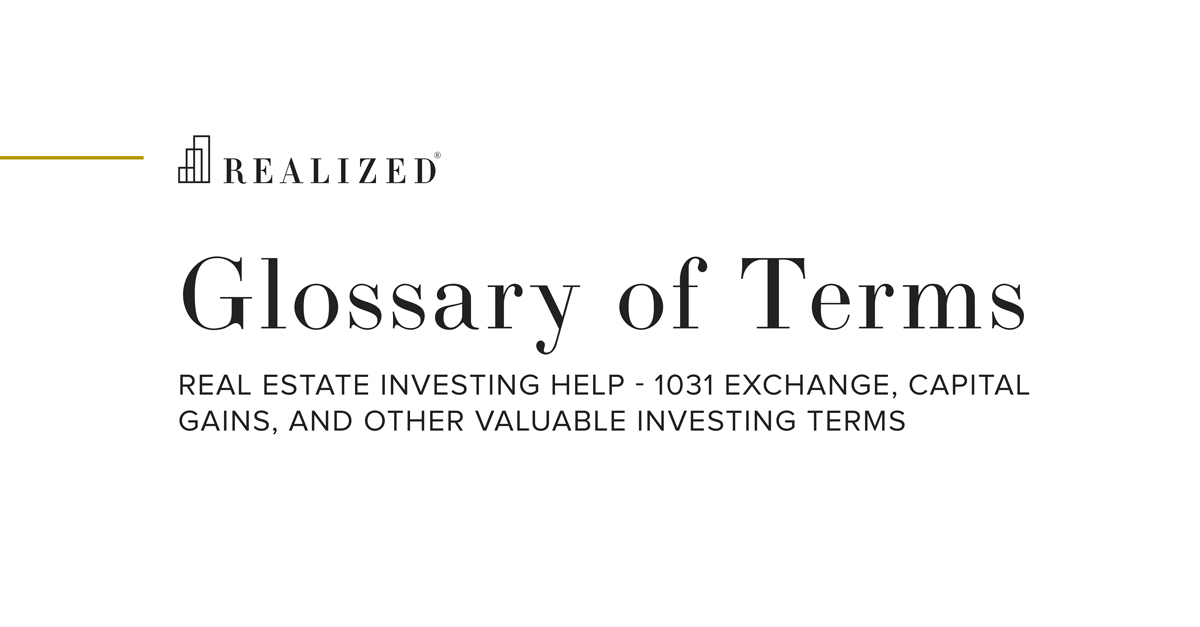Inflation Rate 2019-12-12 08:00:00

Inflation is a percentage measurement of how quickly the price of goods is increasing. It is measured for each country — although regions within a country can experience different rates of inflation. Most countries target 2-3% annual inflation.

To calculate the percent increase of inflation over the last year, take the previous year's Consumer Price Index (CPI) from the current year's index. Divide that number by the previous year's CPI, and multiply that result by 100. To make it simpler, let's call this year's CPI X, and last year's Y:

X - Y / Y x 100 = percent increase of inflation.

There are many factors that can overly increase inflation. When a country prints lots of money, inflation can dramatically increase, as was the cause in Zimbabwe, Africa. To get an idea of how normal inflation affects the prices of goods, the following shows the rising cost of a cup of coffee from 1970-2019:

1970 = \$0.25
1980 = \$0.45
1990 = \$0.75
2000 = \$1.00
2010 = \$1.25
2019 = \$1.59

Source: Investopedia